GeeksforGeeks App
Open AppBrowser
Continue

# Polar Representation of Complex Numbers

We are quite familiar with complex numbers, we know the numbers which are of the form (x + i y) where x & y are real numbers and i (iota) is the imaginary unit. Till now we have mostly discussed the complex numbers of the form (x + i y) this is called the algebraic form or geometrical form of a complex number which is most commonly used but there are some other forms of a complex number too. And we are going to cover another important form of complex numbers that is Polar form or Trigonometrical form.

### Geometrical or Algebraic Form of a Complex Number

The complex number z = (x + i y) is represented by a point P (x, y) on the Argand plane, And every point on the Argand/Complex plane represents a unique complex number. If a complex number is purely real then it’s imaginary part Im(z) = 0 and it lies exactly on the real axis (X-axis), whereas a purely imaginary complex number has its real part Re(z) =0 and it lies exactly on the imaginary axis (Y-axis).

Note: If a point P (x, y) represents a complex number z on the Argand plane then the complex number Z = (x + i y) is known as the affix of the point P.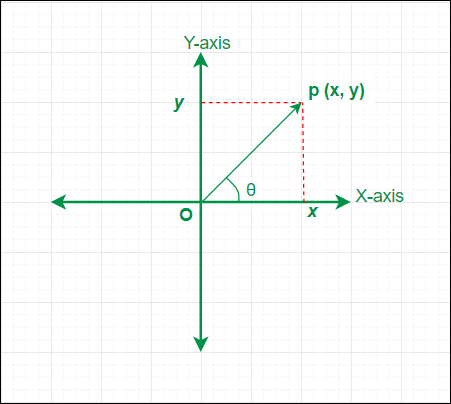Geometrical representation of (z) on Argand plane

In the above representation of the complex number length of the line segment, OP is equal to the modulus of the complex number and the angle θ is the angle formed by OP in the anticlockwise direction with the positive direction of the X-axis known as the amplitude or argument of the complex number denoted by amp(z) or arg(z).

From the above figure, θ can be calculated as follows

tan(θ) = y / x

tan(θ) = |Im(z) / Re(z)|

θ = tan-1{|Im(z) / Re(z)|}

Note: The angle θ can have infinite values in the multiple of 2π. But the unique value of θ that lies in the range (-π ≤ θ ≤ π) is called the principal argument or principal value of the amplitude. The point to be remembered is the value of the principal argument of a complex number (z) depends on the position of the complex number (z) i.e the quadrant in which the point P representing the complex number (z) lies.

Let’s discuss the different cases to find out the value of the principal argument. Let α be the acute angle subtended by OP with the X-axis and θ is the principal argument of the complex number (z).

Case 1. When the complex number z = (x + i y) lies in the first quadrant i.e. x > 0 & y > 0 then the value of the principal argument (θ = α).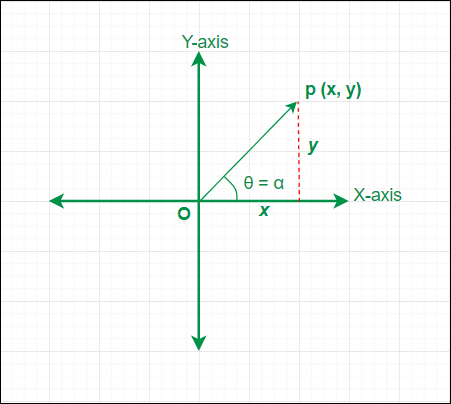Z = (x + i y) in 1st quadrant

Case 2. When the complex number z = (x + i y) lies in the second quadrant i.e. x < 0 & y > 0 then the value of the principal argument (θ = π – α).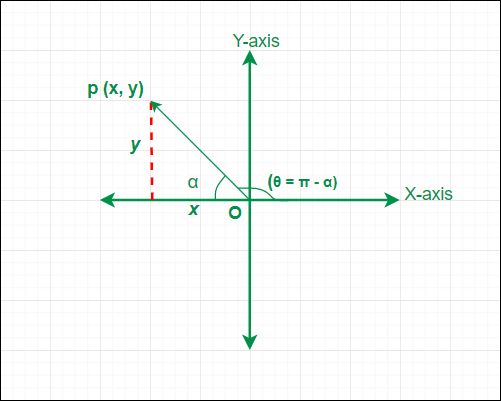Z = (x + i y) in 2nd quadrant

Case 3. When the complex number z = (x + i y) lies in the third quadrant i.e. x < 0 & y < 0 then the value of the principal argument (θ = α – π).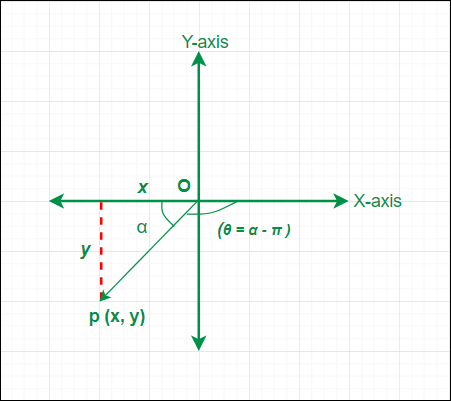Z = (x + i y) in 3rd quadrant

Case 4. When the complex number z = (x + i y) lies in the fourth quadrant i.e. x > 0 & y < 0 then the value of the principal argument (θ = -α).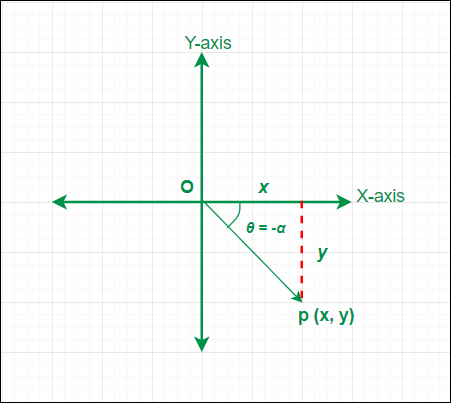Z = (x + i y) in 4th quadrant

### Polar or Trigonometrical Form of a Complex Number

We have seen the geometrical form of a complex number (z) in which it is represented by (x, y) on the Argand plane, OP = |z| and arg(z) = θ. Now we will use the geometrical form of a complex number to obtain its polar form. In the polar or trigonometrical form, the complex number (z) is represented by (r, θ). where r = |z| & θ = arg(z).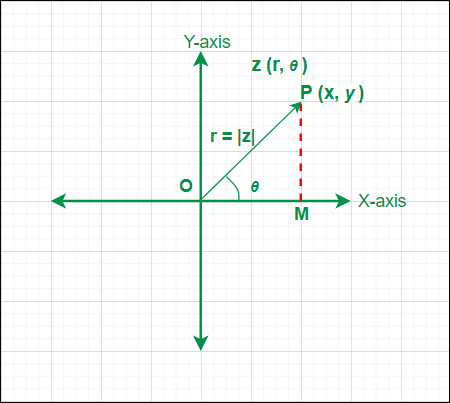The polar form of z (r, θ)

From the above figure, we can find OM = x = |z| cos θ and MP = y = |z| sin θ ;

Now put the values of x & y in z = (x + i y) and we get z = |z| cos θ + i |z| sin θ

Taking |z| common, z = |z| (cos θ + i sin θ)

And putting |z| = r ;

The polar form of a complex number (z) is given by

z = r (cos θ + i sin θ) ;

If we take the general value of the argument arg(z) = 2n π + θ, then the polar form of z is given by

z = r [cos (2n π + θ) + i sin (2n π + θ)] ; where n is an integer.

As we have θ in the expression of the polar form of z then again there will be four different cases depending upon the principal argument values θ  in the four quadrants. Let’s discuss them using the results obtained above for the principal argument θ.

• Case 1. When the complex number z lies in the first quadrant then the value of the principal argument (θ = α). So, the polar form of z = r (cos α + i sin α).
• Case 2. When the complex number z lies in the second quadrant then the value of the principal argument (θ = π – α). So, the polar form of z = r [cos (π – α) + i sin (π – α)] or z = r (-cos α + i sin α)
• Case 3. When the complex number z lies in the third quadrant then the value of the principal argument (θ = α – π). So, the polar form of z = r [cos (α – π) + i sin (α – π)] or z = r (-cos α – i sin α)
• Case 4. When the complex number z lies in the fourth quadrant then the value of the principal argument (θ = -α). So, the polar form of z = r [cos (-α) + i sin (-α)] or z = r (cos α – i sin α).

As we have discussed both the forms of complex numbers and we have also understood that the polar form of a complex number z (r, θ) can easily be obtained from its geometrical/algebraic form z (x, y); Let’s see one sample problem on the conversion of the complex number in the algebraic form to the polar form.

### Examples

Example 1: If (z = -i) or (z = 0 – i) is a complex number in the algebraic form then its polar form representation is given by

Solution:

As z = (0 – i) ≈ P (0, -1), it lies on the Y-axis (θ = -α)

α = tan-1(Im(z) / Re(z))

α = tan-1(|(-1) / 0|) = tan-1(|-∞|) = π / 2

θ = -α = -(π / 2)

r = |z| = √(02 + 12) = 1

z = r (cos θ + i sin θ)

z = 1 [cos(π / 2) – i sin(π / 2)]

Example 2: If z = (1 + i) is a complex number in the algebraic form then its polar form representation is given by

Solution:

As z = (1 + i) ≈ P (1, 1), it lies in the first quadrant then (θ = α)

α = tan-1(Im(z) / Re(z))

α = tan-1(1) = π / 4

θ = α = π / 4

r = |z| = √(12 + 12) = √2

z = r (cos θ + i sin θ)

z = √2 [cos(π / 4) + i sin(π / 4)]

Example 3: If z = (-1 – √3 i) is a complex number in the algebraic form then its polar form representation is given by

Solution:

As z = (-1 – √3 i) ≈ P (-1, -√3), it lies in the third quadrant then (θ = α – π)

α = tan-1(Im(z) / Re(z))

α = tan-1(|-√3 /(-1)|) = tan-1(√3) = π / 3

θ = (α – π)

θ = (π  / 3) – π = – (2 π)  / 3

r = |z| = √((-1)2 + (-√3)2) = √4 = 2

z = r (cos θ + i sin θ)

z = 2 [cos(-(2π) / 3) + i sin((-2π) / 3)]

z = 2 [cos(2π / 3) – i sin(2π / 3)] or

z = 2 [- cos(π / 3) – i sin(π / 3)]

My Personal Notes arrow_drop_up
Related Tutorials